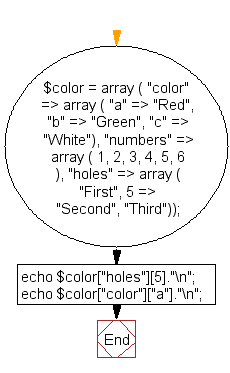﻿ PHP Array Exercise: Print two values from a given array - w3resource# PHP Array Exercises : Print two values from a given array

## PHP Array: Exercise-19 with Solution

Write a PHP script to print "second" and Red from the following array.
Sample Data :
\$color = array ( "color" => array ( "a" => "Red", "b" => "Green", "c" => "White"),
"numbers" => array ( 1, 2, 3, 4, 5, 6 ),
"holes" => array ( "First", 5 => "Second", "Third"));

Sample Solution:

PHP Code:

``````<?php
\$color = array ( "color" => array ( "a" => "Red", "b" => "Green", "c" => "White"),
"numbers" => array ( 1, 2, 3, 4, 5, 6 ),
"holes" => array ( "First", 5 => "Second", "Third"));
echo \$color["holes"]."\n"; // prints "second"
echo \$color["color"]["a"]."\n"; // prints "Red"
?>
```
```

Sample Output:

```Second
Red
```

Flowchart:PHP Code Editor:

What is the difficulty level of this exercise?

﻿

## PHP: Tips of the Day

PHP: Convert one date format into another in PHP

The second parameter to date() needs to be a proper timestamp (seconds since January 1, 1970). You are passing a string, which date() can't recognize.

You can use strtotime() to convert a date string into a timestamp. However, even strtotime() doesn't recognize the y-m-d-h-i-s format.

PHP 5.3 and up

Use DateTime::createFromFormat. It allows you to specify an exact mask - using the date() syntax - to parse incoming string dates with.

PHP 5.2 and lower

You will have to parse the elements (year, month, day, hour, minute, second) manually using substr() and hand the results to mktime() that will build you a timestamp.

But that's a lot of work! I recommend using a different format that strftime() can understand. strftime() understands any date input short of the next time joe will slip on the ice. for example, this works:

```\$old_date = date('l, F d y h:i:s');         // returns Saturday, January 30 10 02:06:34
\$old_date_timestamp = strtotime(\$old_date);
\$new_date = date('Y-m-d H:i:s', \$old_date_timestamp);
```

Ref : https://bit.ly/33QzKPG